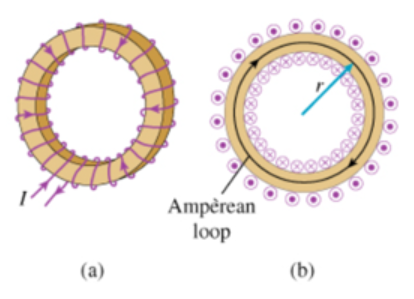# Problem: A toroid is a solenoid bent into the shape of a doughnut. It looks similar to a toy Slinky with ends joined to make a circle. Consider a toroid consisting of N turns of a single wire with current I flowing through.Part A The magnetic field inside the toroid varies as a function of which parameters?  O a) r onlyb) θ only c)  both r and θ In an ideal toroid, current would flow only in the and k directions. The magnetic field in the central plane, outside of the coils of such a toroid, is zero. For the toroid shown in the figures however, this field is not quite zero. This is because in this problem, there is a single wire that is wrapped around a doughnut shape. This wire must point somewhat in the -θ direction, and thus the current must actually have a component in the -θ direction. Part BCompute B, the magnitude of the magnetic field in the center of the toroid, that is, on the z axis in the plane of the toroid. Assume that the toroid has an overall radius of R (the distance from the center of the toroid to the middle of the wire loops) and that R is large compared to the diameter d of the individual turns of the toroid coils.Note that whether the field points upward or downward depends on the direction of the current, that is, on whether the coil is wound clockwise or counterclockwise. Express B in terms of μ, R, I, N, and the local diameter d of the coils.

###### FREE Expert Solution

Ampere's law:

$\overline{){\mathbf{\oint }}{\mathbf{B}}{\mathbf{·}}{\mathbf{d}}{\mathbf{s}}{\mathbf{=}}{{\mathbf{\mu }}}_{{\mathbf{0}}}{{\mathbf{I}}}_{\mathbf{e}\mathbf{n}\mathbf{c}\mathbf{l}\mathbf{o}\mathbf{s}\mathbf{e}\mathbf{d}}}$

Biot-Savart law:

$\overline{){\mathbf{B}}{\mathbf{=}}{\mathbf{\int }}\frac{{\mathbf{\mu }}_{\mathbf{0}}\mathbf{Isin\theta d}\stackrel{\mathbf{⇀}}{\mathbf{l}}\stackrel{\mathbf{^}}{\mathbf{z}}}{\mathbf{4}\mathbf{\pi }{\mathbf{r}}^{\mathbf{2}}}}$

Part A

Suppose the loop has N turns, and ds = 2πr, the magnetic field then becomes:

B2πr = μ0NI

99% (472 ratings)###### Problem Details

A toroid is a solenoid bent into the shape of a doughnut. It looks similar to a toy Slinky with ends joined to make a circle. Consider a toroid consisting of N turns of a single wire with current I flowing through.Part A

The magnetic field inside the toroid varies as a function of which parameters?  O

a) r only

b) θ only

c)  both r and θ

In an ideal toroid, current would flow only in the and k directions. The magnetic field in the central plane, outside of the coils of such a toroid, is zero. For the toroid shown in the figures however, this field is not quite zero. This is because in this problem, there is a single wire that is wrapped around a doughnut shape. This wire must point somewhat in the -θ direction, and thus the current must actually have a component in the -θ direction.

Part B

Compute B, the magnitude of the magnetic field in the center of the toroid, that is, on the z axis in the plane of the toroid. Assume that the toroid has an overall radius of R (the distance from the center of the toroid to the middle of the wire loops) and that R is large compared to the diameter d of the individual turns of the toroid coils.

Note that whether the field points upward or downward depends on the direction of the current, that is, on whether the coil is wound clockwise or counterclockwise. Express B in terms of μ, R, I, N, and the local diameter d of the coils.